##### Chemistry Workbook For Dummies with Online PracticeIn chemistry, you can add and subtract extreme numbers by using exponential notation, and expressing your numbers as coefficients of identical powers of 10. To wrestle your numbers into this form, you may need to use coefficients less than 1 or greater than 10.

To add two numbers by using exponential notation, you begin by expressing each number as a coefficient and a power of 10. In this example, you add these numbers,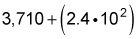by following these steps:

1. Convert both numbers to the same power of 10.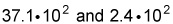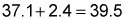3. Join your new coefficient to the shared power of 10.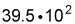## Subtracting with exponential notation

To subtract numbers in exponential notation, you follow the same steps but subtract the coefficients. Here’s an example:

0.0743 – 0.0022

To perform the subtraction, follow these steps:
1. Convert both numbers to the same power of 10.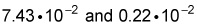2. Subtract the coefficients. 7.43 – 0.22 = 7.21
3. Join your new coefficient to the shared power of 10.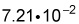Now try a few practice questions.

## Practice questions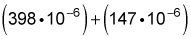2. Use exponential notation to subtract the following: 9,352 – 431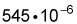Because the numbers are each already expressed with identical powers of 10 (in this case, 10–6), you can simply add the coefficients: 398 + 147 = 545 Then join the new coefficient with the original power of 10.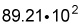(or an equivalent expression). First, convert the numbers so each uses the same power of 10: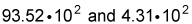Here, you’ve picked 10², but any power is fine as long as the two numbers have the same power. Then subtract the coefficients: 93.52 – 4.31 = 89.21 Finally, join the new coefficient with the shared power of 10.